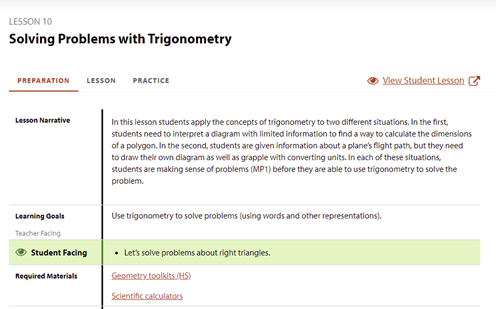Go to website

# Solving problems with trigonometry

In this lesson, students apply the concepts of trigonometry to two different situations. In each of these situations, students are making sense of problems before they use trigonometry to solve the problem.

Year level(s) Year 10
Audience Teacher
Purpose Content knowledge, Teaching resource
Format Web page
Teaching strategies and pedagogical approaches Explicit teaching
Keywords Angles, Angle, Tangent, Cosine, Sine, Unit circle, Quadrant, Coordinate plane

## Curriculum alignment

Curriculum connections Critical and creative thinking
Strand and focus Space
Topics Pythagoras and trigonometry
AC: Mathematics (V9.0) content descriptions
AC9M10M03

Solve practical problems applying Pythagoras' theorem and trigonometry of right-angled triangles, including problems involving direction and angles of elevation and depression

Numeracy progression Understanding geometric properties (P7)
Understanding units of measurement (P10)